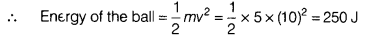# Calculate the energy converted into heat and sound

A ball of mass 5 kg is released from rest at a height of 30 m above the ground. It is travelling with 10 ms-1, when it hit the floor and does not rebounds. If the gravitational field strength is 20 Nkg-1, then calculate the energy converted into heat and sound.

Given, height above the ground, h = 30 m
Mass of the ball, m = 5 kg
Speed of the ball while striking the floor, v = 10 ms-1
Acceleration due to gravity, g = 10 Nkg-1
According to the law of conservation of energy, all the energy of the ball will be converted into heat and sound energy because the ball does not rebound.Hence, 250 J of energy will be converted into heat and energy.